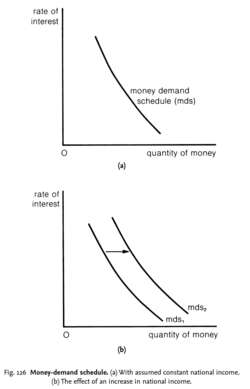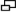money-demand scheduleFig. 126 Money-demand schedule. (a) With assumed constant national income. The effect of an increase in national income.

(b) The effect of an increase in national income.

money-demand schedule

a schedule that depicts the relationship between the quantity of MONEY demanded, Qd (LIQUIDITY PREFERENCE), and the INTEREST RATE, i, at a given level of NATIONAL INCOME, Y:The money-demand schedule has three components: (i) the TRANSACTION DEMAND FOR MONEY (that is, money held on a day-to-day basis to finance current expenditures on goods and services); (ii) the PRECAUTIONARY DEMAND FOR MONEY (that is, money held to cover for unforeseen contingencies); and (iii) the SPECULATIVE DEMAND FOR MONEY (that is, money held to purchase BONDS in anticipation of a fall in their price).

If the rate of interest falls, this increases the speculative demand for money. The rate of interest varies inversely with the price of bonds. For example, if the nominal return on a bond is 10p and the price of the bond is £1, then the effective interest rate is 10%; if the price of the bond falls to 50p, the effective interest rate increases to 20%. Consequently, the lower the rate of interest is, the higher will be the price of bonds. The higher the price of bonds, the less likely it is that bond prices will continue to rise and the greater the chances that they will fall. Thus, as shown in Fig. 126 (a), the lower the rate of interest, the greater is the inducement to hold cash for speculative purposes.

An increase in national income increases the TRANSACTION DEMAND FOR MONEY and may increase the PRECAUTIONARY DEMAND for money, shifting the money demand schedule outwards to the right, from mds1 to mds2 in Fig. 126. The money-demand schedule interacts with the MONEY-SUPPLY SCHEDULE to determine the equilibrium INTEREST RATE.

References in periodicals archive ?
Which of these possibilities occurs depends, in a complicated way, on the second and third derivatives of the money-demand schedule.
Figures 3 and 4 plot qualitatively the modulus of the eigenvalues for this system, computed at the monetary steady state, for the Cagan money-demand schedule.
Bruno and Fischer (1990) interpret a related model with bonds and a Cagan money-demand schedule.

Site: Follow: Share:
Open / Close